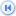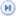Spike's Calculators

# Mass/Weight Units

A glossary of some of the mass/weight units found in the Metric, Troy, Apothecary, and Avoirdupois systems. Includes units that are no longer in use at this time.

Unit NameConversion TypeSystemNoteImperial/USMetric
apothecaries units of massMass/WeightApothecarya system used by apothecaries in weighing out potions and medicines, abbreviated to ap
assay ton (AT UK)Mass/WeightUK unit1 assay ton UK = 32.66667 grams proportionately represents 1 long ton = 32666.666677 troy ounces1 assay ton (AT UK) = 504.1 grains (gr)1 assay ton (AT UK) = 32.66667 grams (g)
assay ton (AT US)Mass/WeightUS Customary1 assay ton US = 29.16667 grams proportionately represents one short ton = 29166.66667 troy ounces1 assay ton (AT US) = 450.1 grains (gr)1 assay ton US = 29.16667 grams (g)
avoirdupois units of massMass/WeightAvoirdupois Avoirdupois is a traditional system of mass/weight in the Imperial and the US Customary systems, abbreviated to advp
carat (ct)Mass/WeightMetrica carat is a unit of mass used for weighing diamonds and other precious stones. One carat is equal to 10 points, 200 milligrams1 carat (ct) = 3.087 grains (gr)1 carat (ct) = 0.2 grams (g)
centigram (cg) Mass/WeightMetricunit of mass equal to 10 milligrams, one hundred of a gram1 centigram (cg) = 0.1543 grains (gr)1 centigram (cg) = 0.01 grams (g)
decagram (dag)Mass/WeightMetricthe decagram a rarely used measure1 decagram (dag) = 154.3 grain (gr)1 decagram (dag) = 10 grams (g)
decigram (dg)Mass/WeightMetricSI unit of mass equal to one tenth of a gram1 decigram (dg) = 1.543 grains (gr)1 decigram (dg) = 0.1 grams (g)
dram (dr advp)Mass/WeightAvoirdupois the avoirdupois dram is 1/16th of an ounce (oz avdp) and 1/256th of a pound (lb avdp)1 dram (dr advp) = 27.344 grains (gr)1 dram (dr advp) = 1.7718 grams (g)
dram (dr ap)Mass/WeightApothecaryUnit in the apothecaries weight system equal to 1/8th ounce (oz ap) and 1/96th pound (lb ap)1 dram (dr ap) = 60 grains (gr)1 dram (dr ap) = 3.8879 grams (g)
gamma (ɣ)Mass/WeightMetric/oldone gamma is equal to one microgram1 gamma (?) = 0.0000154 grains (gr)I gamma (y)= 0.000001 grams (g)
grain (gr)Mass/WeightAvoirdupois/Troy/Apothecarythe grain is the same in avoirdupois, troy, and apothecaries units of mass1 grain (gr) = 0.05 scruple (s ap)1 grain (gr) = 0.0648 grams (g)
gram (g)Mass/WeightMetricSI unit of mass equal to one thousandth of a kilogram.1 gram (g) = 15.43 grains (gr)1 gram = 0.001 kilograms (kg)
hectogram (hg)Mass/WeightMetricSI unit of mass equal to one tenth of a kilogram1 hectogram (hg) = 0.22046 pounds (lb avdp)1 hectogram (hg) = 100 grams (g)
hundredweight (cwt long)Mass/WeightAvoirdupois The long hundred weight is equal to 1/20 long ton1 hundredweight (cwt long) = 112 pounds (lb avdp)1 hundredweight (cwt long) = 50.8 kilograms (kg)
hundredweight (cwt short)Mass/WeightUS CustomaryThe short hundred weight is equal to 1/20 short ton1 hundredweight (cwt short) = 100 pounds (lb avdp)1 hundredweight (cwt short)= 45.359 kilograms (kg)
kilogram (kg)Mass/WeightMetricThe kilogram is a unit of weight, a measure in common usage throughout the world and the base unit in the International System of Units1 kilogram (kg) = 2.2046 pounds (lb avdp)1 kilogram (kg)= 1000 grams (g)
metric unit of massMass/WeightMetricweight system based on the decimal system. The modern name for the metric system is the SI, International System of Units with the kilogram as a mass base unit
microgram (µg) Mass/WeightMetricSI unit of mass equal to one millionth of a gram 1 microgram (µg) = 0.0000154 grains (gr)1 microgram (µg) = 0.000001 grams (g)
micropound (µlb)Mass/WeightAvoirdupois the micropound is a small unit of avoirdupois weight and mass in the US and imperial systems 1 micropound (µlb) = 0.007grains (gr)1 micropound (µlb) = 0.000454 grams (g)
milligram (mg) Mass/WeightMetricSI unit of mass equal to one thousandth of a gram1 milligram (mg) = 0.0154 grains (gr)1 milligram (mg) = 0.001 grams (g)
nanogram (ng) Mass/WeightMetricSI unit of mass equal to one billionth of a gram. 1 nanogram (ng) = 0.0000000154 grains (gr)1 nanogram = 10e-9 grams (g)
ounce (oz advp)Mass/WeightAvoirdupois one ounce (oz advp) is uequal to 1/16th pound (lb avdp)1 ounce (oz advp) = 437.5 grains (gr)1 ounce (oz advp) = 28.3495 grams
ounce (oz ap)Mass/WeightApothecaryone ounce (oz ap) is equal to 1/12th pound (lb ap)1 ounce (oz ap) = 480 grains (gr)1 ounce (oz ap) = 31.103 grams (gr)
ounce (oz tr)Mass/WeightTroyone ounce (oz tr) is equal to 1/12th pound (lb tr)1 ounce (oz tr)= 480 grains (gr)1 ounce tr = 31.103 grams (gr)
pennyweight (dwt) Mass/WeightTroythe pennyweight (dwt ) is a unit of mass equal to , 1/20 th ounce (oz tr) , ?1/240 pound (lb tr)1 pennyweight (dwt) = 24 grains (gr)1 pennyweight (dwt) = 1.555 grams (g)
point (pt)Mass/Weightmetrica unit of mass used for precious stones equalling one tenth of a carat1 point (pt) = 0.03086 grains (gr)1 point (pt) = 0.002 grams (g)
pound (lb ap)Mass/WeightApothecarythis pound is is the largest unit in the apothecaries weight system. one pound (tr and ap) = 12 ounces (tr or ap)1 pound (lb ap) = 5760 grains (gr)1 pound (lb ap) = 373.242 grams (g)
pound (lb avdp)Mass/WeightAvoirdupois unit of mass in the FPS system. The base unit of the avoirdupois, troy, and apothecaries sytems one pound (advp) 16 ounces (advp)1 pound (lb avdp)= 7000 grains (gr)1 pound (lb avdp)= 453.5 grams (g)
pound (lb tr)Mass/WeightTroythe Troy pound is the base unit of the Troy system of weights1 pound (lb tr) =5760 grains (gr)1 pound (lb tr) = 373.242 grams (g)
quarter (qt long)Mass/WeightAvoirdupois quarter (qt long) is based on one quarter of a long hundredweight1 quarter (qt long) = 28 pounds (lb advp)1 quarter (qt long) = 12.7 kilograms kg)
quarter (qt short)Mass/WeightUS Customaryquarter (qt short)based on one quarter of a short hundredweight1 quarter (qt short) = 25 pounds (lb advp)1 quarter short = 11.3398 kilograms (kg)
scruple (s ap)Mass/WeightApothecaryused by apothecaries - scrupulum, a small weight - scrupulus, small stone, pebble, dim of scrupus1 scruple (s ap) = 20 grains (gr)1 scruple (s ap) = 1.296 grams (g)
stone (st)Mass/WeightAvoirdupois traditional British unit of mass/weight equal to 14 avoirdupois pounds which is among others, used for expressing the weight of a person1stone (st) = 14 pounds (lb avdp)1 stone = 6.35 kilograms (kg)
ton (long t)Mass/WeightAvoirdupois long ton is the name off the ton used in the UK and sometimes known as the gross ton1 ton long = 2240 pound (lb avdp)1 ton long = 1016 kilograms (kg)
ton (short tn) Mass/WeightUS Customaryshort ton is the standard ton used with in US Customary measures, sometimes known as the net ton1 ton (short tn) = 2000 pounds (lb avdp)1 ton (short tn) = 907.185 kilograms (kg)
tonne (t)Mass/WeightMetricmetric unit of mass equal to one thousand kilograms known in North America as the metric ton1 tonne (t) = 0.984 ton (long t) and 1 tonne (t)= 1.102 (tn) short1 tonne (t) = 1000 kilograms (kg)
troy units of massMass/WeightTroyEnglish weight system based on a troy pound of 5760 grains,Troy is abbreviated to tr